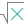#SUBTOTAL (PQL - xl)

This function calculates a subtotal of a list of numbers using a specified built-in function

• Library: PQL \ Spreadsheet \ Math & Trig
• Compatibility: Any content (regardless of data source) in the Tabulate spreadsheet module

#### Syntax

SUBTOTAL(function num, ref1, ref2)

##### Function Arguments
 Name Description Type Optional function num The number that represents the built-in function to use for the subtotal Number ref1 Double-precision floating-point value, an integer value, or an array (cell range) of these StringOrNumber ref2 Double-precision floating-point value, an integer value, or an array (cell range) of these StringOrNumber